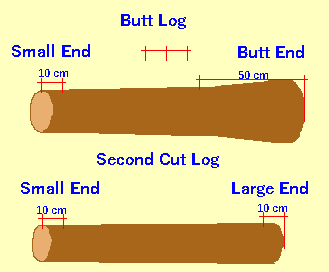Spike's Calculators

# Swedish Log Volume Calculation Method Three

Swedish Log Volume Calculators: Method One - Method Two - Method Three"Measuring of Roundwood" as recommended by the Timber Measurement Council

Measuring log volume using small and large end diameter measurements.
Small end - large end diameter measurement may be used for all tree species on logs with a top diameter of ≥ 3 cm and length ≤ 6.5 m.
The diameter is measured 10 cm from each end of the log, with the exception of the butt end of a butt log, where the measurement is taken 50 cm from the end.

##### Formula used:
`V =  π ÷ 4 * L[cDl² + (1- c)Dt²]`
where:
V = the log volume in cubic metres
c = constant
Dt = small end diameter taken 10 cm from end of log
Dl = large end diameter taken 10 cm from end of log/ taken 50 cm from the end of a butt log

### Using Small and Large Diameters

Length of Log m
Small end diameter cm
Large end diameter cm

#### Results:

 Log Volume m³

#### Calculation

1. the log length in metres
2. top end diameter measured 10 cm in from top end of log in centimetres
3. large end diameter measured 50 cm in on a butt log, 10 cm in on other logs